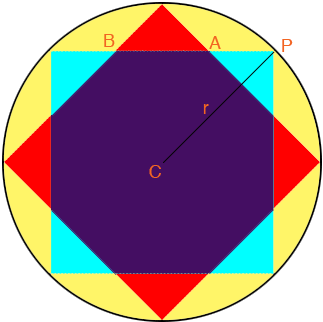SEARCH HOMEMath Central Quandaries & QueriesQuestion from Kermit, a student: How do you find the area of the star that is formed by two squares and surrounded by a circle. The only given information is that the radius of the circle is 10.Hi,

I drew a circle of radius $r = 10$ units and two squares, one red and one blue, inscribed in the circle to form a regular, 8 pointed star.The area of the star is the area of the red square plus the area of the blue star minus the area of the purple regular octagon since it is part of both squares. Can you find the lengths of the sides of the squares so that you can calculate their areas?

If you know the side length of a regular octagon you can find it area using this note or this one. Can you find the side length $|AB|?$

If you have trouble write back, tell us what you have done, and we will try to help.

PennyMath Central is supported by the University of Regina and the Imperial Oil Foundation.